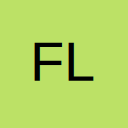# Can Inputbox display a partly dynamic message based on Columns?

I am having a few difficulties with the input box. I want the inputbox to display class number and name relative to the row the input is for, can I somehow acheive this? Also on an exit sub command is it possible to have an are you sure option?

This is where I am at I have tried to comment where I am am stuck.

Edit: having another side issue trying to update this code is that The Next doesn't see the For

• Re: Can Inputbox display a partly dynamic message based on Columns?

This should do what you want for the exit sub

Code
``````Dim iResponce As Integer
iResponce = MsgBox("Are You Sure", vbYesNo, "Exit?")
If iResponce = vbYes Then ' You Clicked YES!
' PUT YES CODE HERE!
Else
' PUT NO CODE HERE!
End If``````

[SIZE=3]The Only Dumb Question Is A Question Not Asked.[/SIZE]

• Re: Can Inputbox display a partly dynamic message based on Columns?

Take this for a spin.

[SIZE=3]The Only Dumb Question Is A Question Not Asked.[/SIZE]

• Re: Can Inputbox display a partly dynamic message based on Columns?

You code has got a little muddled in a few places:

Code
``````ce.FormulaR1C1 = _
"=IF(AND(RC[-19]<>0,((RC[-18]*1.65)+(RC[-17])+(RC[-16]*0.8))<>0, [COLOR=red]_"[/COLOR] ' I don't seem to be able to make a new line here?
[COLOR=red]([/COLOR]RC[-20]-(RC[-20]/((RC[-18]*1.65)+(RC[-17])+(RC[-16]*0.8)))*((RC[-17])+(RC[-16]*0.8)))/RC[-19],0)*1.25"``````

You are in the middle of a string constant, you cannot put a continuation in that way. If you want to break up your string (and I can see why) then you would need to do:

Code
``````ce.FormulaR1C1 = _
"=IF(AND(RC[-19]<>0,((RC[-18]*1.65)+(RC[-17])+(RC[-16]*0.8))<>0," & _
"(RC[-20]-(RC[-20]/((RC[-18]*1.65)+(RC[-17])+(RC[-16]*0.8)))*((RC[-17])+(RC[-16]*0.8)))/RC[-19],0)*1.25"``````

Basically break the string into two separate strings and use concatenation to rejoin them. Your continuation can go after the concatenation.

Also:

Your structure has become mangled, you left the If Then unfinished and started your Select Case. Then the End If has come before the End Select. Compiler got lost.

You seem to want to make a decision on rRange but unless it is defined externally then it will be Nothing at the point where you test it.

• Re: Can Inputbox display a partly dynamic message based on Columns?

I am using the code you posted yegarboy, it works for the input but the prompt doesn't show in the inputbox.

Code
``Prompt = "This Class & RC & 'Name' & RC" ' Supposed to refer to column C a N relatively. Not working``
• Re: Can Inputbox display a partly dynamic message based on Columns?

Reading here regarding Inputboxes http://msdn.microsoft.com/en-u…spx#odc_tentipsvba_topic1 they have setup a prompt as

Code
``Dim strFirstName As String     Dim strLastName As String          ' Get the user's first and last name.     strFirstName = InputBox(Prompt:="Type your first name.", _         Title:="Full Name Demo")``

So I thought I could just add this in.

Code
``````Dim UserPrompt As String

getchoice = InputBox("Enter 0,1 or 2")
UserPrompt = InputBox(Prompt:="This Class" & RC & "Name" & RC)``````

Can't quite get it to work though

Updated it to this but not yet working.

Code
``````getchoice = InputBox("Enter 0,1 or 2")
UserPrompt = InputBox(Prompt:="This Class" & Range("RC") & "Name" & Range("RC"))``````

This displays text but not ranges.

Code
``getchoice = InputBox(Prompt:="Enter 0,1 or 2 This Class & Range(RC) & Name & Range(RC)")``
• Re: Can Inputbox display a partly dynamic message based on Columns?

Is it possible to do what I am trying with the Inputbox message display? Or will i just drive myself nuts tryin• Re: Can Inputbox display a partly dynamic message based on Columns?

The Range method takes the form of:

Code
``````Range("A1")
'or
Range("A" & lRow)``````

Where the variable lRow is dynamic based in something along the lines of

Code
``lRow = cells(rows.count,"A").end(xlup).row``

Change "A" to any applicable column.
Similarly, you can dimension a variable for a dynamic column refrence

Code
``lCol = cells(1,columns.count).end(xltoleft).column``

The reference the dynamic row/column as:

Code
``Range(Cells(lRow,lCol))``

HTH

[COLOR="blue"][SIZE="4"][FONT="Verdana"]AAE[/FONT][/SIZE][/COLOR]
[COLOR="blue"]----------------------------------------------------[/COLOR]

• Re: Can Inputbox display a partly dynamic message based on Columns?

It really does help. Can I ask how can I get the row variable to start from the top. I am using lrow as above and I am shown my last entry.

Would integer be the correct type for lrow because thats what I used.

Code
``Dim lrow As Integer``
• Re: Can Inputbox display a partly dynamic message based on Columns?

Quote

Can I ask how can I get the row variable to start from the top

Not entirely sure what you're after here, but you can use ".end(xlDown)" instead of ".end(xlUp)".
However, if you want the last row in a column (xlDown) will stop at the first blank cell in the column. For the last used row in the column, always use (xlUp).

Quote

Would integer be the correct type for lrow because thats what I used.

Excel converts integers to long type. I would dimension the variable as a long type from the start.

[COLOR="blue"][SIZE="4"][FONT="Verdana"]AAE[/FONT][/SIZE][/COLOR]
[COLOR="blue"]----------------------------------------------------[/COLOR]

• Re: Can Inputbox display a partly dynamic message based on Columns?

Regarding starting variabel from top. The inputbox ranges "lrow" doesn't show the correct class and number in the inputbox. The inputbox displays the last name and number in the list. I want to start from the first name and number in the list and work down. I tried the up and down but didn't seem to change what range was displayed.

## Participate now!

Don’t have an account yet? Register yourself now and be a part of our community!